Request a Free Counselling Session from our Expert Mentor# NCERT Solution for Class 12 Chemistry Chapter 3

Swastik Classes’ NCERT Solutions for Class 12 Chemistry Chapter 3 – Electrochemistry, provide students with a detailed understanding of the concepts of electrochemistry. Our solutions cover topics such as electrochemical cells, Nernst equation, and the different types of electrodes used in electrochemistry.

Our solutions provide step-by-step explanations for all the questions in the chapter, making it easy for students to follow the concepts and learn effectively. We also include diagrams and illustrations to help students visualize the processes and concepts better.

Our NCERT Solutions for Class 12 Chemistry Chapter 3 are designed to help students prepare for their exams and score well. By using our solutions, students can build a strong foundation in electrochemistry and gain a deeper understanding of the subject.

Overall, our NCERT Solutions for Class 12 Chemistry Chapter 3 are an effective resource for students to learn and master the concepts of electrochemistry. Students can use our solutions to prepare for their exams, revise the concepts covered in the chapter, and gain confidence in their understanding of electrochemistry.

## Chemistry Class 12 NCERT solutions chapter 3 intext questions and answers

Question 3.1:

How would you determine the standard electrode potential of the systemMg2+ | Mg?

The standard electrode potential of Mg2+ | Mg can be measured with respect to the standard hydrogen electrode, represented by Pt(s), H2(g) (1 atm) | H+(aq)(1 M).

A cell, consisting of Mg | MgSO4 (aq 1 M) as the anode and the standard hydrogen electrode as the cathode, is set up.

Then, the emf of the cell is measured and this measured emf is the standard electrode potential of the magnesium electrode.

Here,electrode potential for the standard hydrogen electrode is zero.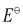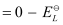Question 3.2:

Can you store copper sulphate solutions in a zinc pot?

Zinc is more reactive than copper. Therefore, zinc can displace copper from its salt solution. If copper sulphate solution is stored in a zinc pot, then zinc will displace copper from the copper sulphate solution.

Hence, copper sulphate solution cannot be stored in a zinc pot.

Question 3.3:

Consult the table of standard electrode potentials and suggest three substances that can oxidise ferrous ions under suitable conditions.

Substances that are stronger oxidising agents than ferrous ions can oxidise ferrous ions.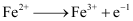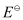= −0.77 V

This implies that the substances having higher reduction potentials than +0.77 V can oxidise ferrous ions to ferric ions. Three substances that can do so are F2, Cl2, and O2.

Question 3.4:

Calculate the potential of hydrogen electrode in contact with a solution whose pH is 10.

For hydrogen electrode,  it is given that pH = 10

∴ [H+] = 10–10 M

Now, using Nernst equation: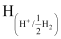= −0.0591 log 1010

= −0.591 V

Note: Since pressure of H2 gas is not mentioned, it is assumed as 1 bar.

Question 3.5:

Calculate the emf of the cell in which the following reaction takes place:

Given that = 1.05 V

Applying Nernst equation we have:

= 1.05 − 0.02955 log 4 × 104

= 1.05 − 0.02955 (log 10000 + log 4)

= 1.05 − 0.02955 (4 + 0.6021)

= 0.914 V

Question 3.6:

The cell in which the following reactions occurs:

has= 0.236 V at 298 K.

Calculate the standard Gibbs energy and the equilibrium constant of the cell reaction.

Here, n = 2,  T = 298 K

We know that:

= −2 × 96487 × 0.236

= −45541.864 J mol−1

= −45.54 kJ mol−1

Again, −2.303RT log Kc

= 7.981

Kc = Antilog (7.981)

= 9.57 × 107

Question 3.7:

Why does the conductivity of a solution decrease with dilution?

The conductivity of a solution is the conductance of ions present in a unit volume of the solution. The number of ions (responsible for carrying current) decreases when the solution is diluted. As a result, the conductivity of a solution decreases with dilution.

Question 3.8:

Suggest a way to determine the value of water.

Applying Kohlrausch’s law of independent migration of ions, the value of water can be determined as follows:

Hence, by knowing the values of HCl, NaOH, and NaCl, the value of water can be determined.

Question 3.9:

The molar conductivity of 0.025 mol L−1 methanoic acid is46.1 S cm2 mol−1.

Calculate its degree of dissociation and dissociation constant.

Given λ°(H+)= 349.6 S cm2 mol−1 and λ °(HCOO−) = 54.6 S cm2 mol

C = 0.025 mol L−1

Now, degree of dissociation:

Thus, dissociation constant:

Question 3.10:

If a current of 0.5 ampere flows through a metallic wire for 2 hours, then how many electrons would flow through the wire?

= 0.5 A

t = 2 hours = 2 × 60 × 60 s = 7200 s

Thus, Q = It

= 0.5 A × 7200 s

= 3600 C

We know that  number of electrons.

Then,

Hence, number of electrons will flow through the wire.

Question 3.11:

Suggest a list of metals that are extracted electrolytically.

Metals that are on the top of the reactivity series such as sodium, potassium, calcium, lithium, magnesium, aluminium are extracted electrolytically.

Question 3.12:

What is the quantity of electricity in coulombs needed to reduce 1 mol of ? Consider the reaction:

The given reaction is as follows:

Therefore, to reduce 1 mole of , the required quantity of electricity will be:

=6 F

= 6 × 96487 C

= 578922 C

Question 3.13:

Write the chemistry of recharging the lead storage battery, highlighting all the materials that are involved during recharging.

A lead storage battery consists of a lead anode, a grid of lead packed with lead oxide (PbO2) as the cathode, and a 38% solution of sulphuric acid (H2SO4) as an electrolyte.

When the battery is in use, the following cell reactions take place:

At anode:

At cathode:

The overall cell reaction is given by,

When a battery is charged, the reverse of all these reactions takes place.

Hence, on charging, PbSO4(s)present at the anode and cathode is converted into Pb(s)and PbO2(s) respectively.

Question 3.14:

Suggest two materials other than hydrogen that can be used as fuels in fuel cells.

Methane and methanol can be used as fuels in fuel cells.

Question 3.15:

Explain how rusting of iron is envisaged as setting up of an electrochemical cell.

In the process of corrosion, due to the presence of air and moisture, oxidation takes place at a particular spot of an object made of iron. That spot behaves as the anode. The reaction at the anode is given by,

Electrons released at the anodic spot move through the metallic object and go to another spot of the object.

There, in the presence of H+ ions, the electrons reduce oxygen. This spot behaves as the cathode. These H+ ions come either from H2CO3, which are formed due to the dissolution of carbon dioxide from air into water or from the dissolution of other acidic oxides from the atmosphere in water.

The reaction corresponding at the cathode is given by,

The overall reaction is:

Also, ferrous ions are further oxidized by atmospheric oxygen to ferric ions. These ferric ions combine with moisture, present in the surroundings, to form hydrated ferric oxidei.e., rust.

Hence, the rusting of iron is envisaged as the setting up of an electrochemical cell.

## NCERT Class 12 Chemistry Chapter 3 Exercise Solutions

Question 3.1:

Arrange the following metals in the order in which they displace each other from the solution of their salts.

Al, Cu, Fe, Mg and Zn

The following is the order in which the given metals displace each other from the solution of their salts.

Mg, Al, Zn, Fe, Cu

*Question 3.2:

Given the standard electrode potentials,

K+/K = −2.93V, Ag+/Ag = 0.80V,Hg2+/Hg = 0.79VMg2+/Mg = −2.37 V,

Cr3+/Cr = − 0.74V

Arrange these metals in their increasing order of reducing power.

The lower the reduction potential, the higher is the reducing power. The given standard electrode potentials increase in the order of K+/K < Mg2+/Mg < Cr3+/Cr < Hg2+/Hg < Ag+/Ag.

Hence, the reducing power of the given metals increases in the following order:Ag < Hg < Cr < Mg <K

Question 3.3:

Depict the galvanic cell in which the reaction Zn(s) + 2Ag+(aq) → Zn2+(aq) + 2Ag(s) takes place. Further show:

(i)  Which of the electrode is negatively charged?

(ii)  The carriers of the current in the cell.

(iii)  Individual reaction at each electrode.

The galvanic cell in which the given reaction takes place is depicted as:

(i)  Zn electrode (anode) is negatively charged.

(ii)  Ions are carriers of current in the cell and in the external circuit, current will flow from silver to zinc.

(iii)  The reaction taking place at the anode is given by,

The reaction taking place at the cathode is given by,

Question 3.4:

Calculate the standard cell potentials of galvanic cells in which the following reactions take place:

(i)  2Cr(s) + 3Cd2+(aq) → 2Cr3+(aq) + 3Cd

(ii)  Fe2+(aq) + Ag+(aq) → Fe3+(aq) + Ag(s)

Calculate the ΔrGθ and equilibrium constant of the reactions.

(i)

The galvanic cell of the given reaction is depicted as:

Now, the standard cell potential is

In the given equation,

n = 6

F = 96487 C mol−1

Then,  = −6 × 96487 C mol−1 × 0.34 V

= −196833.48 CV mol−1

= −196833.48 J mol−1

= −196.83 kJ mol−1

Again,

= −RT ln K

= 34.496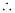K = antilog (34.496)

= 3.13 × 1034

(ii)

The galvanic cell of the given reaction is depicted as:

Now, the standard cell potential is

Here, n = 1.

Then,

= −1 × 96487 C mol−1 × 0.03 V

= −2894.61 J mol−1

= −2.895 kJ mol−1

Again,

= 0.5073K = antilog (0.5073)

= 3.2 (approximately)

*Question 3.5:

Write the Nernst equation and emf of the following cells at 298 K:

(i)  Mg(s) | Mg2+(0.001M) || Cu2+(0.0001 M) | Cu(s)

(ii)  Fe(s) | Fe2+(0.001M) || H+(1M)|H2(g)(1bar) | Pt(s)

(iii)  Sn(s) | Sn2+(0.050 M) || H+(0.020 M) | H2(g) (1 bar) | Pt(s)

(iv)  Pt(s) | Br2(l) | Br(0.010 M) || H+(0.030 M) | H2(g) (1 bar) | Pt(s).

(i)  For the given reaction, the Nernst equation can be given as:

= 2.7 − 0.02955

= 2.67 V (approximately)

(ii)  For the given reaction, the Nernst equation can be given as:

= 0.52865 V

= 0.53 V (approximately)

(iii)  For the given reaction, the Nernst equation can be given as:

= 0.14 − 0.0295 × log125

= 0.14 − 0.062

= 0.078 V

(iv)  For the given reaction, the Nernst equation can be given as:

Question 3.6:

In the button cells widely used in watches and other devices the following reaction takes place:

Zn(s) + Ag2O(s) + H2O(l) → Zn2+(aq) + 2Ag(s) + 2OH(aq)

Determineandfor the reaction.= 1.104 V

We know that,

= −2 × 96487 × 1.04

= −213043.296 J

= −213.04 kJ

*Question 3.7:

Define conductivity and molar conductivity for the solution of an electrolyte. Discuss their variation with concentration.

Conductivity of a solution is defined as the conductance of a solution of 1 cm in length and area of cross-section 1 sq. cm. The inverse of resistivity is called conductivity or specific conductance. It is represented by the symbolκ. If ρ is resistivity, then we can write:

The conductivity of a solution at any given concentration is the conductance (G) of one unit volume of solution kept between two platinum electrodes with the unit area of cross-section and at a distance of unit length.

i.e.,

(Since a = 1, l = 1)

Conductivity always decreases with a decrease in concentration, both for weak and strong electrolytes. This is because the number of ions per unit volume that carry the current in a solution decreases with a decrease in concentration.

Molar conductivity:

Molar conductivity of a solution at a given concentration is the conductance of volume V of a solution containing 1 mole of the electrolyte kept between two electrodes with the area of cross-section A and distance of unit length.

Now, l = 1 and A = V (volume containing 1 mole of the electrolyte).

Molar conductivity increases with a decrease in concentration. This is because the total volume V of the solution containing one mole of the electrolyte increases on dilution.

The variation of withfor strong and weak electrolytes is shown in the following plot:

A is representing strong electrolyte while B is weak electrolyte

Question 3.8:

The conductivity of 0.20 M solution of KCl at 298 K is 0.0248 Scm−1. Calculate its molar conductivity.

Given,

κ = 0.0248 S cm−1

c = 0.20 MMolar conductivity,

= 124 Scm2mol−1

*Question 3.9:

The resistance of a conductivity cell containing 0.001M KCl solution at 298 K is 1500 Ω. What is the cell constant if conductivity of 0.001M KCl solution at 298 K is 0.146 × 10−3 S cm−1.

Given,

Conductivity, κ = 0.146 × 10−3 S cm−1

Resistance, R = 1500 ΩCell constant = κ × R

= 0.146 × 10−3 × 1500

= 0.219 cm−1

*Question 3.10:

The conductivity of sodium chloride at 298 K has been determined at different concentrations and the results are given below:

Concentration/M 0.001 0.010 0.020 0.050 0.100

102 × κ/S m−1  1.237 11.85 23.15 55.53 106.74

Calculate for all concentrations and draw a plot between and c½. Find the value of

Given,

κ = 1.237 × 10−2 S m−1, c = 0.001 M

Then, κ = 1.237 × 10−4 S cm−1, c½ = 0.0316 M1/2

= 123.7 S cm2 mol−1

Given,

κ = 11.85 × 10−2 S m−1, c = 0.010M

Then, κ = 11.85 × 10−4 S cm−1, c½ = 0.1 M1/2

= 118.5 S cm2 mol−1

Given,

κ = 23.15 × 10−2 S m−1, c = 0.020 M

Then, κ = 23.15 × 10−4 S cm−1, c1/2 = 0.1414 M1/2

= 115.8 S cm2 mol−1

Given,

κ = 55.53 × 10−2 S m−1, c = 0.050 M

Then, κ = 55.53 × 10−4 S cm−1, c1/2 = 0.2236 M1/2

= 111.1 1 S cm2 mol−1

Given,

κ = 106.74 × 10−2 S m−1, c = 0.100 M

Then, κ = 106.74 × 10−4 S cm−1, c1/2 = 0.3162 M1/2

= 106.74 S cm2 mol−1

Now, we have the following data:

Since the line interrupts  at 124.0 S cm2 mol−1, = 124.0 S cm2 mol−1.

Question 3.11:

Conductivity of 0.00241 M acetic acid is 7.896 × 10−5 S cm−1. Calculate its molar conductivity and if for acetic acid is 390.5 S cm2 mol−1, what is its dissociation constant?

Given, κ = 7.896 × 10−5 S m−1

c = 0.00241 mol L−1

Then, molar conductivity,

= 32.76S cm2 mol−1

Again,  = 390.5 S cm2 mol−1

Now,

= 0.084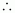Dissociation constant,

= 1.86 × 10−5 mol L−1

Question 3.12:

How much charge is required for the following reductions

(i)  1 mol of Al3+ to Al.

(ii)  1 mol of Cu2+ to Cu.

(iii)  1 mol of  to Mn2+.

(i)Required charge = 3 F

= 3 × 96487 C

= 289461 C

(ii)

Required charge = 2 F

= 2 × 96487 C

= 192974 C

(iii)

i.e.,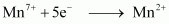Required charge = 5 F

= 5 × 96487 C

= 482435 C

Question 3.13:

How much electricity in terms of Faraday is required to produce

(i)  20.0 g of Ca from molten CaCl2.

(ii)  40.0 g of Al from molten Al2O3.

(i)  According to the question,

Electricity required to produce 40 g of calcium = 2 F

Therefore, electricity required to produce 20 g of calcium= 1 F

(ii)  According to the question,

Electricity required to produce 27 g of Al = 3 F

Therefore, electricity required to produce 40 g of Al = 4.44 F

*Question 3.14:

How much electricity is required in coulomb for the oxidation of

(i)  1 mol of H2O to O2.

(ii)  1 mol of FeO to Fe2O3.

(i)  According to the question,

Now, we can write:

Electricity required for the oxidation of 1 mol of H2O to O2 = 2 F

= 2 × 96487 C

= 192974 C

(ii)  According to the question,

Electricity required for the oxidation of 1 mol of FeO to Fe2O3 = 1 F

= 96487 C

Question 3.15:

A solution of Ni(NO3)2 is electrolysed between platinum electrodes using a current of 5 amperes for 20 minutes. What mass of Ni is deposited at the cathode?

Given,Current = 5A

Time = 20 × 60 = 1200 s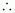Charge = current × time

= 5 × 1200

= 6000 C

According to the reaction,

Nickel deposited by 2 × 96487 C = 58.71 g

Therefore, nickel deposited by 6000 C = 1.825 g

Hence, 1.825 g of nickel will be deposited at the cathode.

Question 3.16:

Three electrolytic cells A,B,C containing solutions of ZnSO4, AgNO3 and CuSO4, respectively are connected in series. A steady current of 1.5 amperes was passed through them until 1.45 g of silver deposited at the cathode of cell B. How long did the current flow? What mass of copper and zinc were deposited?

According to the reaction:

i.e., 108 g of Ag is deposited by 96487 C.

Therefore, 1.45 g of Ag is deposited by

= 1295.43 C

Given,Current = 1.5 ATime

= 863.6 s

= 864 s

= 14.40 min

Again,

i.e., 2 × 96487 C of charge deposit = 63.5 g of Cu

Therefore, 1295.43 C of charge will deposit = 0.426 g of Cu

i.e., 2 × 96487 C of charge deposit = 65.4 g of Zn

Therefore, 1295.43 C of charge will deposit = 0.439 g of Zn

*Question 3.17:

Using the standard electrode potentials given in Table 3.1, predict if the reaction between the following is feasible:

(i)  Fe3+(aq) and I(aq)

(ii)  Ag+ (aq) and Cu(s)

(iii)  Fe3+ (aq) and Br− (aq)

(iv)  Ag(s) and Fe3+ (aq)

(v)  Br(aq) and Fe2+ (aq).

Since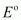for the overall reaction is positive, the reaction between Fe3+(aq) and I(aq) is feasible.

Sincefor the overall reaction is positive, the reaction between Ag+ (aq) and Cu(s) is feasible.

Sincefor the overall reaction is negative, the reaction between Fe3+(aq) and Br(aq) is not feasible.

Sincefor the overall reaction is negative, the reaction between Ag (s) and Fe3+ (aq) is not feasible.

Sincefor the overall reaction is positive, the reaction between Br2(aq) and Fe2+(aq) is feasible.

*Question 3.18:

Predict the products of electrolysis in each of the following:

(i)  An aqueous solution of AgNO3 with silver electrodes.

(ii)  An aqueous solution of AgNO3with platinum electrodes.

(iii)  A dilute solution of H2SO4with platinum electrodes.

(iv)  An aqueous solution of CuCl2 with platinum electrodes.

(i)  At cathode:

The following reduction reactions compete to take place at the cathode.

The reaction with a higher value ofE0 takes place at the cathode. Therefore, deposition of silver will take place at the cathode.

At anode:

The Ag anode is attacked by ions. Therefore, the silver electrode at the anode dissolves in the solution to form Ag+.

(ii)  At cathode:

The following reduction reactions compete to take place at the cathode.

The reaction with a higher value oftakes place at the cathode. Therefore, deposition of silver will take place at the cathode.

At anode:

Since Pt electrodes are inert, the anode is not attacked by  ions. Therefore, OH or  ions can be oxidized at the anode. But OH ions having a lower discharge potential and get preference and decompose to liberate O2.

(iii)  At the cathode, the following reduction reaction occurs to produce H2 gas.

At the anode, the following processes are possible.

For dilute sulphuric acid, reaction (i) is preferred to produce O2 gas. But for concentrated sulphuric acid, reaction (ii) occurs.

(iv)  At cathode:

The following reduction reactions compete to take place at the cathode.

The reaction with a higher value oftakes place at the cathode. Therefore, deposition of copper will take place at the cathode.

At anode: The following oxidation reactions are possible at the anode.

At the anode, the reaction with a lower value ofis preferred. But due to the over-potential of oxygen, Cl gets oxidized at the anode to produce Cl2 gas.

## Topics to study in NCERT chemistry class 12 chapter 3 – Electrochemistry

 Section Number Topics 3.1 Electrochemical Cells 3.2 Galvanic Cells 3.2.1 Measurement of Electrode Potential 3.3 Nernst Equation 3.3.1 Equilibrium Constant from Nernst Equation 3.3.2 Electrochemical Cell and Gibbs Energy of Reaction 3.4 The conductance of Electrolytic Solutions 3.4.1 Measurement of the Conductivity of Ionic Solutions 3.4.2 Variation of Conductivity and Molar Conductivity with Concentration 3.5 Electrolytic Cells and Electrolysis 3.5.1 Products of Electrolysis 3.6 Batteries 3.6.1 Primary Batteries 3.6.2 Secondary Batteries 3.7 Fuel Cells 3.8 Corrosion

## Weightage of Chemistry class 12 chapter 3

This chapter is going to give you 4 marks in your CBSE Term I exam. You can grab all 4 marks by practicing questions and learning concepts. By this, you will be able to solve the objective types of questions in your exam in a very short period of time.

 Chapter Marks Electrochemistry 4 Marks

## Conclusion

Swastik Classes’ NCERT Solutions for Class 12 Chemistry Chapter 3 – Electrochemistry, provide comprehensive and detailed explanations for all the questions in the chapter. Our solutions cover all the important topics in electrochemistry, including electrochemical cells, Nernst equation, and various types of electrodes.

Our solutions are designed to help students learn and understand the concepts of electrochemistry in a clear and concise manner. We provide step-by-step explanations, diagrams, and illustrations to make the learning process easy and effective.

Using our solutions, students can prepare for their exams, revise the concepts covered in the chapter, and strengthen their understanding of electrochemistry. Our solutions can also be used as a reference guide for students who need additional help or clarification on any particular topic.

Overall, Swastik Classes’ NCERT Solutions for Class 12 Chemistry Chapter 3 are an excellent resource for students who want to excel in their exams and build a strong foundation in electrochemistry.

## Why do students follow SWC’s Chemistry class 12 solutions for chapter 3?

At SWC, students directly learn from IITians and experienced faculties. We provide quality study materials to prepare and follow a LEAP model. We teach not only for exams but for lifelong applications.## Is NCERT enough for solid-state JEE?

In the NCERT textbook, every topic is covered in detail. You can easily score well in your Exam by following NCERT thoroughly. You can find some questions in jee that came directly from the NCERT book and some concepts-related questions. Many JEE Toppers and experts also said to follow NCERT for JEE and other related Exams. It covers most concepts and gives more value quickly than reading many reference books available in the market. You can use reference books as a supplement to understand critical topics given in NCERT. Meanwhile, NCERT textbooks are enough for solid-state JEE, and experts explain this.

## FAQs related to NCERT chemistry class 12 chapter 3

### What is electrochemistry class 12th?

Electrochemistry is the branch of chemistry that deals with studying the production of electrical energy from chemical energy released during a chemical reaction. In simple, this is the relation between electrical energy and chemical energy.

### What is an electrolyte in chemistry?

An electrolyte is a substance that conducts electricity by dissociating ions ( Anions and cations ) in the solution. Sodium, calcium, potassium, chlorine, magnesium, and phosphate are the few electrolytes.

### What is electrolysis?

When electric current passes through the electrolytes, the solution’s ionic substances are broken down into simpler forms to conduct electricity. This process is known as electrolysis.

### What are cathode and anode?

A cathode is an electrode where reduction occurs and is negatively charged. An anode is an electrode where oxidation occurs and is positively charged. The key difference between cathode and anode is given below.

 Cathode Anode At negative side At positive side Electricity flows out of the cathode Electricity moves into the anode Electron donor Electron acceptor The cathode can become anode in a galvanic cell The anode can become cathode in a galvanic cell

### What are the factors affecting Electrolytic Conductance?

Following are the factors which affect Electrolytic conductance:

• Interionic Interactions
• Solvation of Ions
• Viscosity of Solvent
• Temperature
• Concentration of Solution